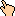## 1998-04 Solution

Pick a number from 1 through 9.
Let's call this number k and keep track, algebraicly, of what the results are.
Subtract 5 from the number (negative results are ok)
k - 5
Multiply the result by 3.
3k - 15
Square the result.
9k2 - 90k + 225 = 9 (k2 - 10k + 25)
Add the digits of the result until there's only one digit. (Example: 64. 6+4=10. 1+0=1.)
This is the same as taking the previous result mod 9. But the previous result was divisible by 9 so here we either have 0 or 9, regardless of what k was.
If the result is less than 5, add 5; if not, subtract 4.
If 0 we get 5, and if it was 9 we get 5, so we have 5 here.
Multiply the result by 2.
10
Subtract 6 from the result.
4
Locate the corresponding letter in the alphabet. (Examples: 1=A, 2=B.)
d
Pick a country that begins with that letter.
Most people pick Denmark, but other options include Dominican Republic
Note the second letter in that country's name. Think of a mammal that begins with that letter.
If Denmark, E, so we could choose elephant or emu. If Dominican Republic, O, so orangutan or octopus.
Now think of the color of that mammal. What do you have?
Most will have chosen a grey elephant from Denmark.

WWW Maven: Dan Garcia (ddgarcia@cs.berkeley.edu)Send me feedback